# A great algorithm summary that has evolved computersByKai Schreiber

The speed of IT technology evolution is spectacular, but what supports italgorithmThis is a processing method (technical idea) called. Among various algorithms, the great algorithms that are said to have revolutionized the evolution of computers are as follows.

Great Algorithms that Revolutionized Computing
http://en.docsity.com/news/interesting-facts/great-algorithms-revolutionized-computing/

Huffman code(Compression algorithm)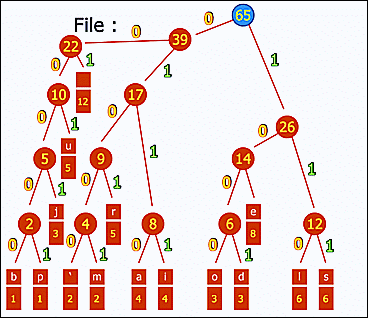Huffman coding (Huffman code)Is an algorithm developed by David Huffmann in 1951. Considering a tournament tree that fights depending on the frequency of frequent occurrence, by giving codes of 0 and 1 for each block, short codes are given for frequent frequencies and long codes are given for frequent frequencies . Huffman codes that can be computed simply and quickly are used for compression techniques such as JPEG and MP3.

Public key cryptography(Encryption algorithm)ByRichard-G

Encryption that enhances confidentiality of communication has the risk of eavesdropping in the process of delivering keys to be decrypted. It solved this problemPublic key cryptographySo we prepared two kinds of encryption keys, "public key" which is not problematic even if it is eavesdropped, not only the "secret key", but also eliminating the eavesdropping risk. In addition to encrypting the plain text with the public key, it is also possible to perform encryption that can be decrypted with the public key with the secret key, and since it can prove "the fact that it has a secret key" it is also used for digital signature .

Dijkstra method(Search algorithm)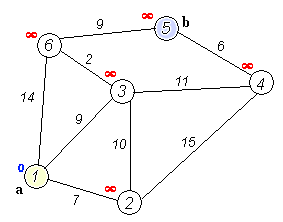Dijkstra methodWas invented by Edgar Dijkstra in 1956 as an algorithm to search for the shortest path. The biggest advantage of the Dijkstra method is that "it can omit the calculation after that when it is found to be an unnecessary route." The Dijkstra method is used for route guidance of car navigation systems.

Binary search(Search algorithm)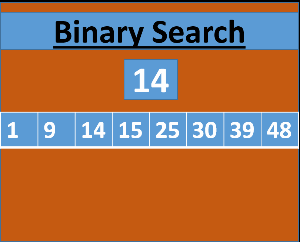Binary searchIs an algorithm that can efficiently reach the object while narrowing down the search range by searching while sorting an ordered list into two, and it is applied to telephone directory search technology and others.

Quick sort(Alignment algorithm)Quick sortIs an algorithm developed by Mr. Tony Hoa in 1960 and became famous by being adopted as UNIX default alignment function. The greatest feature is its processing speed. Quick sorting is an algorithm with very few data comparisons and exchanges, so you can sort randomly scattered data efficiently and it is evaluated as the fastest sorting algorithm for practical use.

Karatsuba law(Multiplication algorithm)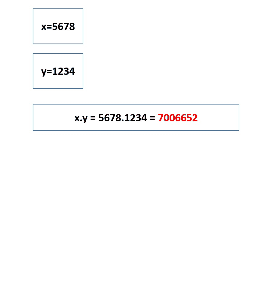In multiplication (multiplication) of a large number, an algorithm that increases the number of additions / subtractions (adding and subtracting) and can multiply the number of multiplication by three quartersKaratsuba lawis. The calculation speed (processing speed) is much greater for addition and subtraction than for multiplication, so it is a miso that can increase the calculation speed in total.

Euclid's parity methodIt is known as the world's oldest algorithm explicitly stated in 300 BCEuclid's parity method. This algorithm, which can quickly find out the greatest common divisor of two natural numbers with simple division and addition, is an active algorithm that is utilized in modern computer technology, such as being used for calculations for public key cryptography.

Bresenham's algorithm(Drawing algorithm)Bresenham's algorithmIs an algorithm developed by IBM's Jack Elton Bresenham in 1962 that is used to draw straight lines on a computer screen and can also draw a circle by extending it. Bresenham's algorithm is a simple method that only uses integer addition and subtraction and bit shifting, so it can be used on many computers and it can be said to be a revolutionary algorithm supporting the early period of computer graphics. Furthermore, due to its high speed and simplicity, it is also being introduced to modern graphic boards.

Fast inverse square root calculation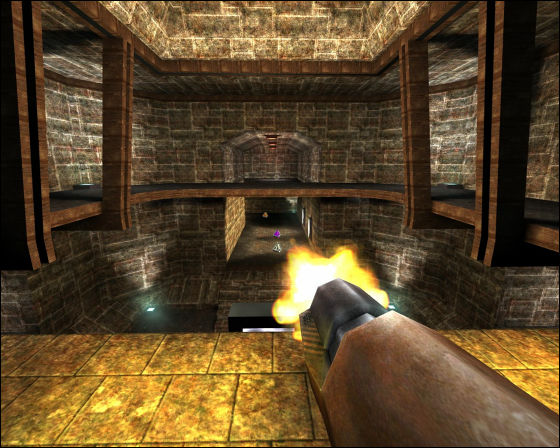High-speed inverse square root calculation algorithm (Fast Inverse Square Root) Is an algorithm adopted in FPS game called Quake III Arena released in 1999, it can calculate reflection of light in 3D graphics at high speed, it is utilized in a situation where speed is required rather than accuracy It is.

in Note,   Software,   Science, Posted by darkhorse_log